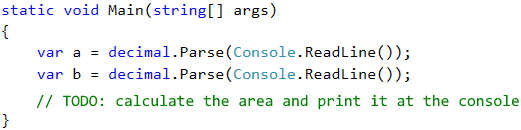# Problem: Calculate Rectangle Area

Write a C# program that reads from the console two numbers, a and b, calculates and prints the area of a rectangle with sides a and b.

a b area
2 7 14
7 8 56
12 5 60

## Video: Problem "Rectangle Area"

Watch a video lesson to learn how to solve the "Rectangle Area" problem step by step: https://youtu.be/6fwNJ5k9zTE.

## Hints and Guidelines

Create a new console C# program. To read both of numbers, use the following commands:What remains is to finish the program above, to calculate the area of the rectangle and to print it. Use the command that is already known to us Console.WriteLine() and put inside its brackets the multiplication of the numbers a and b. In programming, multiplication is done using the operator *.

Test your solution with a few examples. You have to get an output, similar to this one (we enter 2 and 7 as input and the program prints result 14 – their multiplication):

2
7
14